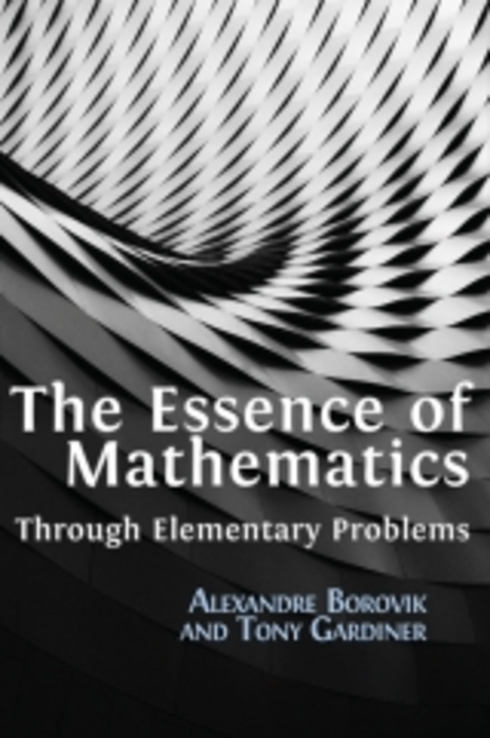# The Essence of Mathematics Through Elementary Problems

(0 reviews)

No ratings

Alexandre Borovik, University of Manchester

Tony Gardiner, University of Birmingham

ISBN 13: 9781783747016

Publisher: Open Book Publishers

Language: English

## Conditions of UseAttribution
CC BY

I. Mental Skills

• 1.1 Mental arithmetic and algebra
• 1.2 Direct and inverse procedures
• 1.3 Structural arithmetic
• 1.4 Pythagoras' Theorem
• 1.5 Visualisation
• 1.7 Regular polygons and regular polyhedra
• 1.8 Chapter 1: Comments and solutions

II. Arithmetic

• 2.1 Place value and decimals: basic structure
• 2.2 Order and factors
• 2.3 Standard written algorithms
• 2.4 Divisibility tests
• 2.5 Sequences
• 2.6 Commutative, associative and distributive laws
• 2.7 Infinite decimal expansions
• 2.8 The binary numeral system
• 2.9 The Prime Number Theorem
• 2.10 Chapter 2: Comments and solutions

III. Word Problems

• 3.1 Twenty problems which embody "3 - 1 = 2"
• 3.2 Some classical examples
• 3.3 Speed and acceleration
• 3.4 Hidden connections
• 3.5 Chapter 3: Comments and solutions

IV. Algebra

• 4.1 Simultaneous linear equations and symmetry
• 4.2 Inequalities and modulus
• 4.3 Factors, roots, polynomials and surds
• 4.4 Complex numbers
• 4.5 Cubic equations
• 4.6 An extra
• 4.7 Chapter 4: Comments and solutions

V. Geometry

• 5.1 Comparing geometry and arithmetic
• 5.2 Euclidean geometry: a brief summary
• 5.3 Areas, lengths and angles
• 5.4 Regular and semi-regular tilings in the plane
• 5.5 Ruler and compasses constructions for regular polygons
• 5.6 Regular and semi-regular polyhedra
• 5.7 The Sine Rule and the Cosine Rule
• 5.8 Circular arcs and circular sectors
• 5.9 Convexity
• 5.10 Pythagoras' Theorem in three dimensions
• 5.11 Loci and coonic sections
• 5.12 Cubes in higher dimensions
• 5.13 Chapter 5: Comments and solutions

VI. Infinity: recursions, induction, infinite descent

• 6.1 Proof by mathematical induction I
• 6.2 'Mathematical induction' and 'scientific induction'
• 6.3 Proof by mathematical induction II
• 6.4 Infinite geometric series
• 6.5 Some classical inequalities
• 6.6 The harmonic series
• 6.7 Induction in geometry, combinatorics and number theory
• 6.8 Two problems
• 6.9 Infinite descent
• 6.10 Chapter 6: Comments and solutions

## Ancillary Material

• Submit ancillary resource

It is increasingly clear that the shapes of reality – whether of the natural world, or of the built environment – are in some profound sense mathematical. Therefore it would benefit students and educated adults to understand what makes mathematics itself ‘tick’, and to appreciate why its shapes, patterns and formulae provide us with precisely the language we need to make sense of the world around us. The second part of this challenge may require some specialist experience, but the authors of this book concentrate on the first part, and explore the extent to which elementary mathematics allows us all to understand something of the nature of mathematics from the inside.

The Essence of Mathematics consists of a sequence of 270 problems – with commentary and full solutions. The reader is assumed to have a reasonable grasp of school mathematics. More importantly, s/he should want to understand something of mathematics beyond the classroom, and be willing to engage with (and to reflect upon) challenging problems that highlight the essence of the discipline.

The book consists of six chapters of increasing sophistication (Mental Skills; Arithmetic; Word Problems; Algebra; Geometry; Infinity), with interleaved commentary. The content will appeal to students considering further study of mathematics at university, teachers of mathematics at age 14-18, and anyone who wants to see what this kind of elementary content has to tell us about how mathematics really works.